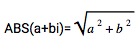# IMABS

Returns the absolute value of a complex number.

### Sample Usage

`IMABS("3+4i")`

`IMABS("6j")`

### Syntax

`IMABS(number)`

• `number` - The complex number to calculate the absolute value of.

### Notes

• The absolute value (or modulus) is defined as follows:
•3 `=IMABS("3+4i")` 5
4 `=IMABS(COMPLEX(3, 4, "j"))` 5